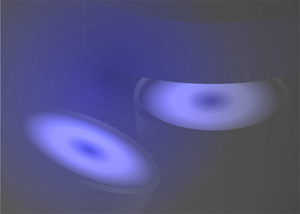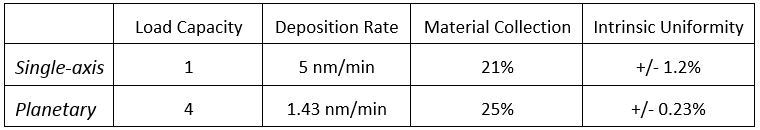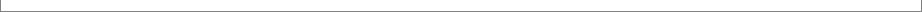Vapor-Collection Efficiency | How to estimate it

​Material-collection efficiency is a fundamental measure of a PVD coating system - it is the material vapor captured by substrates (or substrate carriers) in percentile of the total vapor emitted by a source. For example, a material-collection efficiency of 40% means that for each gram of material removed (evaporated or sputtered) from the source, 0.4 gram is deposited onto the substrate carrier(s); the rest is wasted, unless recycled.As important as it is, quantification of the material collection is not always straightforward. Often, one would like to know the material-collection efficiency before a system is built or modified. What methods are available for us to quantify the material collection? Here we can name three:

1) For systems with simple geometry, analytical formula can provide the answers;
2) Empirical data, with the help of scaling rules, can give estimates of the values; and
3) Numerical computation.

Of the three methods, numerical computation is the most versatile and reliable. Advanced numerical modeling can provide material collection for complex deposition processes which may involve planetary rotation, planet tilt, tilt of sputtering sources, correction masks, and various vapor plume distributions. Modeling software, such as V-Grade 5S, does so by computing the material vapor that lands on the substrate carriers and then dividing it by the total vapor emanated into the hemisphere from a source.

Material-collection efficiency is closely related to deposition rate. V-Grade 5 series software yields deposition rate in units of "nanometer per gram of source material vaporized", which is readily converted to unit "nanometer / minute" in any untested configurations, if its value in one configuration is known - assuming the source is operated under the same power.

To illustrate the utility of such computation, let us consider one scenario. Assume that a magnetron sputtering source provides a deposition rate of 5 nm/min on substrates held by a 30-cm diameter fixture that undergoes a simple rotation; the throw distance is 15 cm; the target is tilted by 18 degrees. According to numerical modeling, assuming a heart-shaped plume function for the vapor flux, the best intrinsic uniformity across the fixture is around +/-1.2% for a material-collection efficiency of 21%. Now, if we want to coat 4 such fixtures that undergo a planetary rotation, what can we expect from the same source? Based on modeling, if the source is placed at a throw distance of 22 cm and is tilted by 17 degrees, we can obtain a deposition rate of 1.43 nm/min and a material collection efficiency of 25%; the intrinsic uniformity is around +/-0.23%. The table below summarizes the main performance measures of the two coating arrangements. Additional analysis on the pros and cons of these two coating arrangements can be found in Examples given on Tin Model website.It should be noted that these values of material collection and deposition rate are the "theoretical" bests by assuming unity sticking coefficient (on substrates) for material particles. Frequently, sticking coefficient is less than 1 due to a number of factors including substrate heating, electron and ion bombardment, particularly when unbalanced magnetrons are employed. Numerical modeling, nevertheless, tells what material-collection efficiency can be achieved in an ideal condition.

© 2018 Tin Model LLC, 2285 Massachusetts Ave.
Cambridge, Massachusetts 02140, U.S.A.
Phone: (857)498-9723
www.tinmodel.com